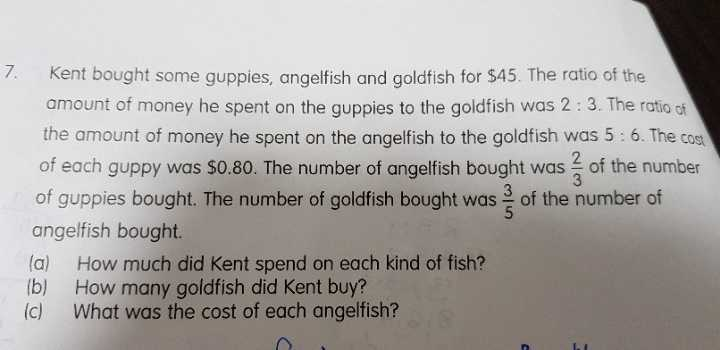# QuestionPls help me with this Q. Thanks

1 Answer

# Answer

By value

Gu : Go       A  :  Go      Total

2    : 3           5  :  6         15

|       |

4  :   6

15u = 45

u = 45 ÷ 15

= 3

a) Amt spent on Guppies = 4 ×\$3

= \$12  (ans)

Amt spent on Gold fish = 6 ×\$3

= \$18 (ans)

Amt spent on Angel fish = \$45-\$12-\$18

=\$15 (ans)

By number

A  :  Gu       Go  :  A

2  :   3           3  :  5

|       |          |      |

10  : 15        6   :   10

Gu: 15u ×\$0.80 = \$12

u = 12 ÷0.8÷15

= 1

b)Number of goldfish = 6u

= 6 (ans)

C)number of angelfish = 10u

= 10

10 angelfish –> \$15

1 angelfish –> \$15÷10

=\$1.50 (ans)

0 Replies 0 Likes